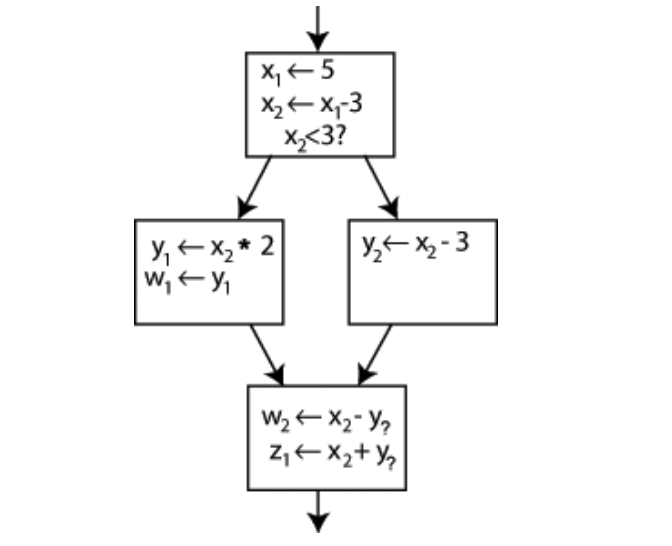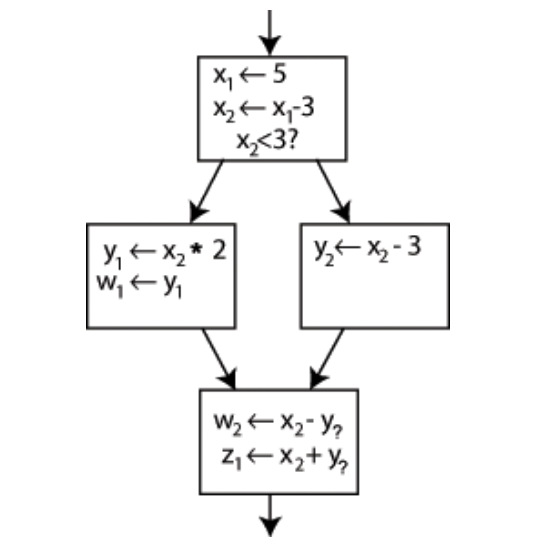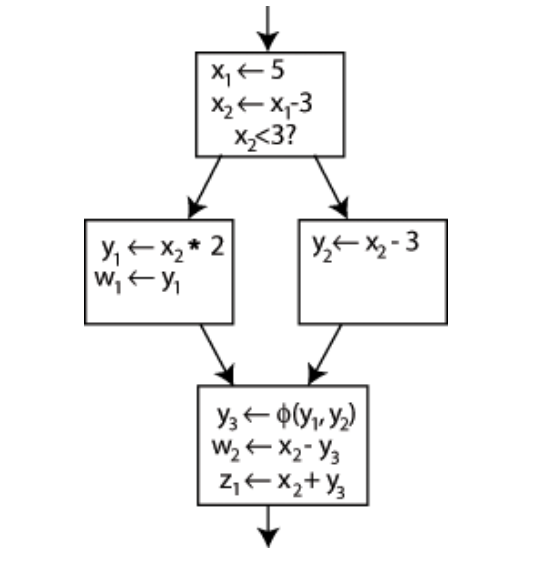×

Search anything:

# LLVM: IR, Assembly, SSA

#### Compiler DesignThis is an introduction to LLVM intermediate code which is low level but still human-readable along with Assembly and Static Single Assignment (SSA).

1. Introduction.
2. LLVM Assembly Code.
3. Intermediate Code.
4. Static Single Assignment - SSA.
5. Summary.
6. References.

## Introduction.

Creating a hello world program and compiling it to binary code;

``````\$ gcc hello.c -o hello.bin; ./hello.bin
``````

A .bin file holds the hello world code in binary format, 0s and 1s. We can view its contents using the hexdump command.

## LLVM Assembly Code.

#### 1. hello world.

We have the following code - test.ll that prints 'Hello world' as output;

``````define i32 @main(){
; hello
call i32 @putchar(i32 72)
call i32 @putchar(i32 101)
call i32 @putchar(i32 108)
call i32 @putchar(i32 108)
call i32 @putchar(i32 111)

; space
call i32 @putchar(i32 32)

; world
call i32 @putchar(i32 119)
call i32 @putchar(i32 111)
call i32 @putchar(i32 114)
call i32 @putchar(i32 108)
call i32 @putchar(i32 100)

; new line
call i32 @putchar(i32 10)

ret i32 0
}

declare i32 @putchar(i32)
``````

i32 - 32-bit integer
The main function is the main entry point to the program
We finally return a value using ret statement.
We use putchar which prints a character passed to it. In this case, we are passing ASCII 32-bit integers which are a representation of characters.

To run the code we first have to compile it to binary code - 0s and 1s then execute it.

For this we execute the following commands;

``````\$ llc-11 -filetype=obj test.ll -o test.o; clang test.o -o test; ./test
``````

Above we first convert it to object code, then use the clang compiler to compile it to binary, and finally, we execute the code.

The contents of a binary code look like the following;

``````00041b0 0000 0000 0000 0000 001b 0000 0001 0000
00041c0 0002 0000 0000 0000 02a8 0000 0000 0000
00041d0 02a8 0000 0000 0000 001c 0000 0000 0000
00041e0 0000 0000 0000 0000 0001 0000 0000 0000
00041f0 0000 0000 0000 0000 0023 0000 0007 0000
0004200 0002 0000 0000 0000 02c4 0000 0000 0000
0004210 02c4 0000 0000 0000 0024 0000 0000 0000
0004220 0000 0000 0000 0000 0004 0000 0000 0000
0004230 0000 0000 0000 0000 0036 0000 0007 0000
0004240 0002 0000 0000 0000 02e8 0000 0000 0000
0004250 02e8 0000 0000 0000 0020 0000 0000 0000
0004260 0000 0000 0000 0000 0004 0000 0000 0000
0004270 0000 0000 0000 0000 0044 0000 fff6 6fff
0004280 0002 0000 0000 0000 0308 0000 0000 0000
``````

We have printed hello world above.

To view the binary code we execute the following command;

``````\$ hexdump hello.bc
``````

### 2. loops.

The following code prints the character 'A' infinitely;

``````; infinite loop
define i32 @main(){
br label %loop
loop:
call i32 @putchar(i32 65)
br label %loop
}

declare i32 @putchar(i32)
``````

Compilation and execution;

``````\$ llc-11 -filetype=obj loop.ll -o loop.o; clang loop.o -o loop; ./loop
``````

### 3. nested Loops.

``````; nested loop
define i32 @main(){
entry:
br label %outer_loop

outer_loop:
%i = phi i32 [0, %entry], [%ii, %end_inner_loop]
br label %inner_loop

inner_loop:
%j = phi i32 [0, %outer_loop], [%jj, %inner_loop]

call i32 @putchar(i32 42) ; print 'A'
%jj = add i32 %j, 1
%inner_cmp_result = icmp sge i32 %jj, 10
br i1 %inner_cmp_result, label %end_inner_loop, label %inner_loop

end_inner_loop:
call i32 @putchar(i32 10) ; print 'w'
%ii = add i32 %i, 1
%outer_cmp_result = icmp sge i32 %ii, 10
br i1 %outer_cmp_result, label %end_outer_loop, label %outer_loop

end_outer_loop:
ret i32 0
}

declare i32 @putchar(i32)
``````

We execute the above loop using the following command;

``````\$ llc-11 -filetype=obj nested.ll -o nested.o; clang nested.o -o nested; ./nested
``````

### 4. If-statements.

``````@.str = private unnamed_addr constant [3 x i8] c"10\00", align 1

define dso_local i32 @main() #0 {
%1 = alloca i32, align 4
%2 = alloca i32, align 4
store i32 0, i32* %1, align 4
store i32 8, i32* %2, align 4
%3 = load i32, i32* %2, align 4
%4 = icmp sgt i32 %3, 6
br i1 %4, label %5, label %7

5:                                                ; preds = %0
%6 = call i32 (i8*, ...) @printf(i8* getelementptr inbounds ([3 x i8], [3 x i8]* @.str, i64 0, i64 0))
store i32 1, i32* %1, align 4
br label %8

7:                                                ; preds = %0
store i32 0, i32* %1, align 4
br label %8

8:                                                ; preds = %7, %5
%9 = load i32, i32* %1, align 4
ret i32 %9
}

declare dso_local i32 @printf(i8*, ...) #1
``````

The above code is equivalent to the following C code;

``````#include<stdio.h>

int main(){
int x = 8;

if(x > 6){
printf("10");
return 1;
}

return 0;
}
``````

We compile and execute by writing;

``````\$ llc-11 -filetype=obj if_statement.ll -o if_statement.o; clang if_statement.o -o if_statement; ./if_statement
``````

To obtain the llvm code from C code we execute the command;

``````\$ clang -emit-llvm -S if_statement.c -o if_statement.ll
``````

## Intermediate Code.

Above, the commands convert code written in the C programming language into .ll files which contain the intermediate representation for the C source code.

### What is clang?

clang is a compiler front-end for programming languages such as Objective C, C, C++. It is intended as a replacement for the GNU Compiler Collection - GCC.
Clang operates in tandem with the LLVM compiler.
Clang is a much faster compiler compared to gcc, in that, the machine code it produces is highly optimized and fast. Also, the code generated uses far less memory.

We use clang compiler which emits code in llvm which is in binary form hence the .bc file extension.
We then disassemble the code and return the human-readable llvm code which is in a file with .ll file extension.

The command compiles C code into human-readable IR.

``````\$ clang -emit-llvm -S multiply.c -o multiply.ll
``````

For this, we need to have installed clang. We install clang by writing:

We have the following LLVM intermediate code;

``````@.str = private unnamed_addr constant [13 x i8] c"Hello world!\00", align 1

; Function Attrs: noinline nounwind optnone uwtable
define dso_local i32 @main() #0 {
%1 = call i32 (i8*, ...)
@printf(i8* getelementptr inbounds
([13 x i8], [13 x i8]* @.str, i64 0, i64 0))
ret i32 0
}

declare dso_local i32 @printf(i8*, ...) #1
``````

Now, to convert the LLVM IR to assembly code we execute the following command:

``````\$ llc hello.bc –o hello.s
``````

Concatenate the assembly code;

The above code is finally converted to binary code that can be executed by the processor.

``````\$ llc-11 -filetype=obj hello.ll -o hello.o; clang hello.o -o hello; ./hello
``````

## Static Single Assignment - SSA.

SSA(Static Single Assignment form) is a property of an IR whereby each variable can only be assigned once, and every variable is defined before its use.
LLVM uses SSA for register values in the primary code representation.
SSA allows for a lot of compiler optimizations such as constant propagation, dead code elimination, global value numbering, value range propagation,, etc. Therefore it is the de-facto standard for IRs in the compilers for imperative programming languages.

An example of SSA and Non-SSA form for if statements;``````x = 5
x = x - 3
if x < 3 then
y = x * 2
w = y
else
y = x - 3
end
w = x - y
z = x + y
``````

Now in SSA form;``````x1 = 5
x2 = x1 - 3
if x2 < 3 the
y1 = x2 * 2
w1 = y1
else
y2 = x2 - 3
end
w2 = x2 - y?
z1 = x2 + y?
``````

The phi - ϕ function is intended to choose between y1 and y2 correctly.``````y3 = phi (y1, y2)
w2 = x2 - y3
z1 = x2 + y3
``````

## Summary.

SSA (Static Single Assignment form) is a property of an IR whereby each variable can only be assigned once, and every variable is defined before its use.
Clang is a much faster compiler compared to gcc, in that, the machine code it produces is highly optimized and fast. Also, the code generated uses far less memory.

Clang operates in tandem with the LLVM compiler.

With this article at OpenGenus, you must have the code idea of IR, Assembly, SSA in LLVM.

#### Erick Lumunge

Erick is a passionate programmer with a computer science background who loves to learn about and use code to impact lives positively.

LLVM: IR, Assembly, SSA